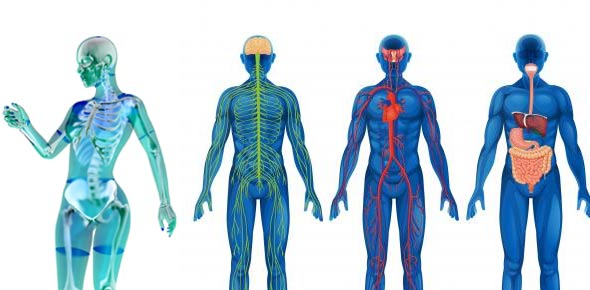# Vnsg Anatomy & Physiology Study Guide

135 Questions | Attempts: 100SettingsHCCS VNSG 1320 Study Guide

• 1.
The study of how the body functions is called:
• A.

Physiology

• B.

Homeostasis

• C.

Anatomy

• D.

Dissection

• 2.
The word derived from 2 Greek words meaning "a cutting up" is:
• A.

Physiology

• B.

Homeostasis

• C.

Anatomy

• D.

Dissection

• 3.
The correct sequence of the level of organization is:
• A.

Cellular, chemical, tissue, organ

• B.

Chemical, cellular, tissue, organ

• C.

Chemical, cellular, organ, tissue

• D.

Chemical, tissue, cellular, organ

• 4.
The smallest living unit of structure is considered to be at the:
• A.

Chemical level

• B.

Cellular level

• C.

Organ level

• D.

Tissue level

• 5.
The reference position for all body directional terms is the:
• A.

Anatomical position

• B.

Prone position

• C.

Supine position

• D.

Sitting position

• 6.
The relationship between the knee and the ankle can be described as:
• A.

The knee is inferior to the ankle

• B.

The knee is distal to the ankle

• C.

The knee is proximal to the ankle

• D.

Both a and b above

• 7.
The relationship between the heart and the lungs can be described as:
• A.

The heart is distal to the lungs

• B.

The heart is medial to the lungs

• C.

The heart is lateral to the lungs

• D.

Both a and c above

• 8.
The term most opposite proximal is:
• A.

Medial

• B.

Superior

• C.

Anterior

• D.

Distal

• 9.
Because humans walk in an upright position, the two terms that can be used interchangeably are:
• A.

Posterior and ventral

• B.

Posterior and inferior

• C.

Posterior and superficial

• D.

Posterior and dorsal

• 10.
The term most opposite medial is:
• A.

Dorsal

• B.

Lateral

• C.

Superficial

• D.

None of the above

• 11.
The relationship between the skin and the muscles can be described as:
• A.

The skin is superficial to the muscle

• B.

The muscle is superficial to the skin

• C.

The muscle is deep to the skin

• D.

Both a and c above

• 12.
A cut dividing the body into anterior and posterior portions is called a:
• A.

Sagittal section

• B.

Frontal section

• C.

Transverse section

• D.

None of the above

• 13.
A cut dividing the body into upper and lower portions is called a:
• A.

Sagittal section

• B.

Frontal section

• C.

Transverse section

• D.

Coronal section

• 14.
A cut dividing the body into right and left portions is called a:
• A.

Sagittal section

• B.

Frontal section

• C.

Transverse section

• D.

Coronal section

• 15.
The mediastinum is part of the:
• A.

Dorsal cavity

• B.

Ventral cavity

• C.

Abdominal cavity

• D.

Both b and c above

• 16.
The 2 major cavities of the body are the:
• A.

Dorsal and ventral

• B.

Thoracic and abdominal

• C.

Pleural and mediastinum

• D.

None of the above

• 17.
The lungs are located in the
• A.

Thoracic cavity

• B.

Mediastinum

• C.

Dorsal cavity

• D.

Both b and c above

• 18.
The word supine describes:
• A.

The body lying face downward

• B.

An anatomical direction

• C.

The reference position of the body

• D.

The body lying face upward

• 19.
Which of the following is not a subatomic particle that makes up the atom?
• A.

Ion

• B.

Proton

• C.

Neutron

• D.

Electron

• 20.
The atomic number of an atom is equal to the number of:
• A.

Electrons

• B.

Neutrons

• C.

Protons

• D.

Both b and c above

• 21.
The atomic mass of an atom is equal to the number of:
• A.

Electrons

• B.

Neutrons

• C.

Protons

• D.

Both b and c above

• 22.
This subatomic particle is found in the nucleus of the atom.
• A.

Electron

• B.

Neutron

• C.

Proton

• D.

Both b and c above

• 23.
This subatomic particle is found in orbitals around the nucleus of the atom.
• A.

Electron

• B.

Neutron

• C.

Proton

• D.

Both b and c above

• 24.
This is not a characteristic of a proton:
• A.

Contributes to the atom's atomic number

• B.

Contributes to the atom's atomic mass

• C.

Is located in the nucleus of the atom

• D.

Carries a negative electrical charge

• 25.
This is not a characteristic of a neutron:
• A.

Contributes to the atom's atomic number

• B.

Contributes to the atom's atomic mass

• C.

Is located in the nucleus of the atom

• D.

Has no electrical charge

## Related TopicsBack to top
×

Wait!
Here's an interesting quiz for you.